## Analogy

#### Analogy

Direction: Select the related figure from the given alternatives.

1. Find the question mark ? figure from answer figure.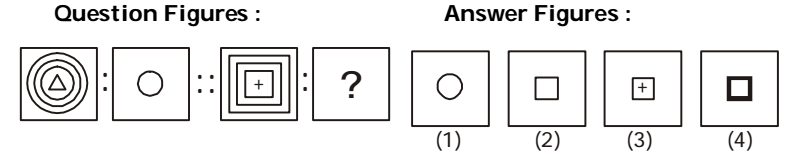1. From first figure to second figure the first, the second and the inner most designs are deleted.

##### Correct Option: B

From first figure to second figure the first, the second and the inner most designs are deleted.

1. Find the question mark ? figure from answer figure.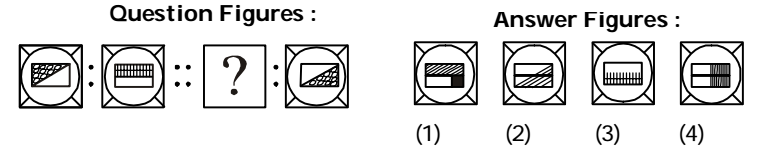1. From first figure to second figure in the first unit of Problem Figures the pattern of design is changed and the oblique line becomes horizontal.

##### Correct Option: C

From first figure to second figure in the first unit of Problem Figures the pattern of design is changed and the oblique line becomes horizontal.

1. Find the question mark ? figure from answer figure.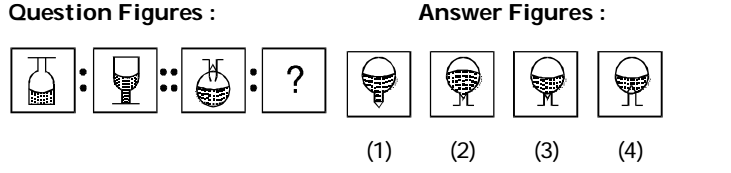1. The second figure is the upside down image of the first figure and the shaded portion moves to the opposite side.

##### Correct Option: C

The second figure is the upside down image of the first figure and the shaded portion moves to the opposite side.

1. Find the question mark ? figure from answer figure.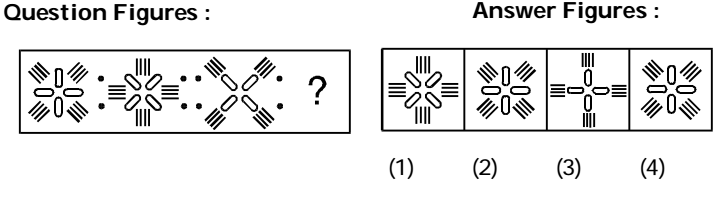1. From first figure to second figure entire design rotates through 45°.

##### Correct Option: C

From first figure to second figure entire design rotates through 45°.

1. Find the question mark ? figure from answer figure.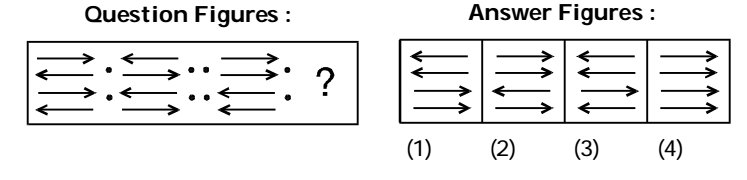1. From first figure to second figure all the arrows are reversed. Alternatively, the second figure is the mirror image of the first figure.

##### Correct Option: A

From first figure to second figure all the arrows are reversed. Alternatively, the second figure is the mirror image of the first figure.